1.3 Vacuum energy

The cosmological constant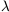is a dimensionful parameter with units of (length)-2. From the point of view of classical general relativity, there is no preferred choice for what the length scale defined bymight be. Particle physics, however, brings a different perspective to the question. The cosmological constant turns out to be a measure of the energy density of the vacuum - the state of lowest energy - and although we cannot calculate the vacuum energy with any confidence, this identification allows us to consider the scales of various contributions to the cosmological constant [14, 15].

Consider a single scalar field, with potential energy V(). The action can be written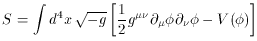(10)

(where g is the determinant of the metric tensor gµ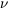), and the corresponding energy-momentum tensor is(11)

In this theory, the configuration with the lowest energy density (if it exists) will be one in which there is no contribution from kinetic or gradient energy, implying ðµ= 0, for which Tµ= - V(0) gµ, where0 is the value ofwhich minimizes V(). There is no reason in principle why V(0) should vanish. The vacuum energy-momentum tensor can thus be written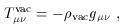(12)

withvac in this example given by V(0). (This form for the vacuum energy-momentum tensor can also be argued for on the more general grounds that it is the only Lorentz-invariant form for Tvacµ.) The vacuum can therefore be thought of as a perfect fluid as in (4), with(13)

The effect of an energy-momentum tensor of the form (12) is equivalent to that of a cosmological constant, as can be seen by moving thegµterm in (7) to the right-hand side and setting(14)

This equivalence is the origin of the identification of the cosmological constant with the energy of the vacuum. In what follows, I will use the terms ``vacuum energy" and ``cosmological constant" essentially interchangeably.

It is not necessary to introduce scalar fields to obtain a nonzero vacuum energy. The action for general relativity in the presence of a ``bare'' cosmological constant0 is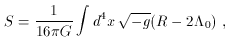(15)

where R is the Ricci scalar. Extremizing this action (augmented by suitable matter terms) leads to the equations (7). Thus, the cosmological constant can be thought of as simply a constant term in the Lagrange density of the theory. Indeed, (15) is the most general covariant action we can construct out of the metric and its first and second derivatives, and is therefore a natural starting point for a theory of gravity.

Classically, then, the effective cosmological constant is the sum of a bare term0 and the potential energy V(), where the latter may change with time as the universe passes through different phases. Quantum mechanics adds another contribution, from the zero-point energies associated with vacuum fluctuations. Consider a simple harmonic oscillator, i.e. a particle moving in a one-dimensional potential of the form V(x) = 1/22 x2. Classically, the ``vacuum'' for this system is the state in which the particle is motionless and at the minimum of the potential (x = 0), for which the energy in this case vanishes. Quantum-mechanically, however, the uncertainty principle forbids us from isolating the particle both in position and momentum, and we find that the lowest energy state has an energy E0 = 1/2(where I have temporarily re-introduced explicit factors offor clarity). Of course, in the absence of gravity either system actually has a vacuum energy which is completely arbitrary; we could add any constant to the potential (including, for example, -1/2) without changing the theory. It is important, however, that the zero-point energy depends on the system, in this case on the frequency.

A precisely analogous situation holds in field theory. A (free) quantum field can be thought of as a collection of an infinite number of harmonic oscillators in momentum space. Formally, the zero-point energy of such an infinite collection will be infinite. (See [10, 3] for further details.) If, however, we discard the very high-momentum modes on the grounds that we trust our theory only up to a certain ultraviolet momentum cutoff kmax, we find that the resulting energy density is of the form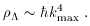(16)

This answer could have been guessed by dimensional analysis; the numerical constants which have been neglected will depend on the precise theory under consideration. Again, in the absence of gravity this energy has no effect, and is traditionally discarded (by a process known as ``normal-ordering''). However, gravity does exist, and the actual value of the vacuum energy has important consequences. (And the vacuum fluctuations themselves are very real, as evidenced by the Casimir effect .)

The net cosmological constant, from this point of view, is the sum of a number of apparently disparate contributions, including potential energies from scalar fields and zero-point fluctuations of each field theory degree of freedom, as well as a bare cosmological constant0. Unlike the last of these, in the first two cases we can at least make educated guesses at the magnitudes. In the Weinberg-Salam electroweak model, the phases of broken and unbroken symmetry are distinguished by a potential energy difference of approximately MEW ~ 200 GeV (where 1 GeV = 1.6 x 10-33 erg); the universe is in the broken-symmetry phase during our current low-temperature epoch, and is believed to have been in the symmetric phase at sufficiently high temperatures in the early universe. The effective cosmological constant is therefore different in the two epochs; absent some form of prearrangement, we would naturally expect a contribution to the vacuum energy today of order(17)

Similar contributions can arise even without invoking ``fundamental" scalar fields. In the strong interactions, chiral symmetry is believed to be broken by a nonzero expectation value of the quark bilinear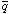q (which is itself a scalar, although constructed from fermions). In this case the energy difference between the symmetric and broken phases is of order the QCD scale MQCD ~ 0.3 GeV, and we would expect a corresponding contribution to the vacuum energy of order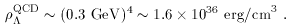(18)

These contributions are joined by those from any number of unknown phase transitions in the early universe, such as a possible contribution from grand unification of order MGUT ~ 1016 GeV. In the case of vacuum fluctuations, we should choose our cutoff at the energy past which we no longer trust our field theory. If we are confident that we can use ordinary quantum field theory all the way up to the Planck scale MPl = (8G)-1/2 ~ 1018 GeV, we expect a contribution of order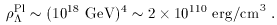(19)

Field theory may fail earlier, although quantum gravity is the only reason we have to believe it will fail at any specific scale.

As we will discuss later, cosmological observations imply(20)

much smaller than any of the individual effects listed above. The ratio of (19) to (20) is the origin of the famous discrepancy of 120 orders of magnitude between the theoretical and observational values of the cosmological constant. There is no obstacle to imagining that all of the large and apparently unrelated contributions listed add together, with different signs, to produce a net cosmological constant consistent with the limit (20), other than the fact that it seems ridiculous. We know of no special symmetry which could enforce a vanishing vacuum energy while remaining consistent with the known laws of physics; this conundrum is the ``cosmological constant problem''. In section 4 we will discuss a number of issues related to this puzzle, which at this point remains one of the most significant unsolved problems in fundamental physics.